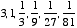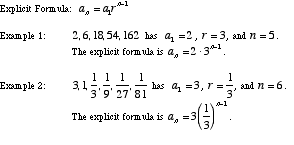index: click on a letter A B C D E F G H I J K L M N O P Q R S T U V W X Y Z A to Z index index: subject areas numbers & symbols sets, logic, proofs geometry algebra trigonometry advanced algebra & pre-calculus calculus advanced topics probability & statistics real world applications multimedia entrieswww.mathwords.com about mathwords website feedback

 Geometric Sequence Geometric Progression A sequence such as 2, 6, 18, 54, 162 orwhich has a constant ratio between terms. The first term is a1, the common ratio is r, and the number of terms is n.See also